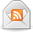# How to Calculate Simple Interest

Posted by Don on March 10, 2015

I get many questions about how to calculate interest. If you are trying to figure out simple interest, the calculation is, well, simple – no pun intended. If you want to calculate compound interest, that gets a little bit more involved. I’ll be writing about compound interest soon, so be sure to keep an eye out for that post. But for now, let’s focus on simple interest.

### What is Simple Interest?

Simple interest is the most basic way you earn interest on your money. It is interest that is earned on the principal alone. Some loans also charge interest based on simple interest. In the case of a loan, simple interest is interest charged on the principal alone. Here is the formula for simple interest:

P x I x N = Simple Interest

P = loan amount
I = interest rate
N = time or number of periods

### Examples of Simple Interest

Let’s look at a few examples to make sure you understand how it works. Let’s say I borrow \$1,000 from the bank at an interest rate of 10% for one year. The calculation would look like this:
P = 1,000
I = 10%
N = 1

1,000 x .10 x 1 = \$100

My interest charge is \$100. Now let’s use the same example, but this time, I borrow the money for five years:

P = 1,000
I = 10%
N = 5

1,000 x .10 x 5 = \$500

In this case, the interest I am charged is \$500. In most cases, you won’t owe the bank the entire interest charge at the end of the loan. The bank will want the interest paid back to them on a monthly basis. So taking the \$100 interest that I owe the bank for the one year loan, we would divide the loan by 12 months to figure out the monthly payment. In this case, it would be \$83.33 of principal and \$8.33 of interest for a total monthly payment of \$91.66.

If you have a savings account in which you earn simple interest on, the calculation would be the same. If you opened the account with \$1,000 that is the amount you earn interest on. The only difference is that instead of owing the bank the interest, the bank owes you the interest.

### Compound or Simple Interest?

When looking at deposit products, you want to seek out those that pay compound interest as opposed to simple interest. The reason is because with compound interest, the interest compounds upon itself and grows faster. I’ll explain this concept more in my compound interest post.

When it comes to loans, many loans work off of simple interest while many more work on the daily accrual method of interest. I am not aware of any loans that use compound interest. If you know of any or come across any, let me know in the comments below.

### Final Thoughts

Overall, calculating simple interest is a fairly easy and straightforward calculation to perform. When you are earning money, compound interest is better than simple interest, simple interest is better than no interest at all. I hope you found this math lesson on simple interest easy to follow and now understand what simple interest is all about.

### More Financial Calculations